# So many graphs!

Algebra Level 4

Consider a quadratic expression in $x$ having real roots $\alpha_{1}$, $\alpha_{2}$ such that they both are within the interval $(-(\alpha_{1}+\alpha_{2}),(\alpha_{1}+\alpha_{2}))$, then enter the numbers of the graphs which are plausible for the expression on $y$-axis and $x$ on the $x$-axis.

Concatenate the numbers in ascending order. For example, if graphs 1,3 and 2 are plausible, enter 123.

$\large 1.$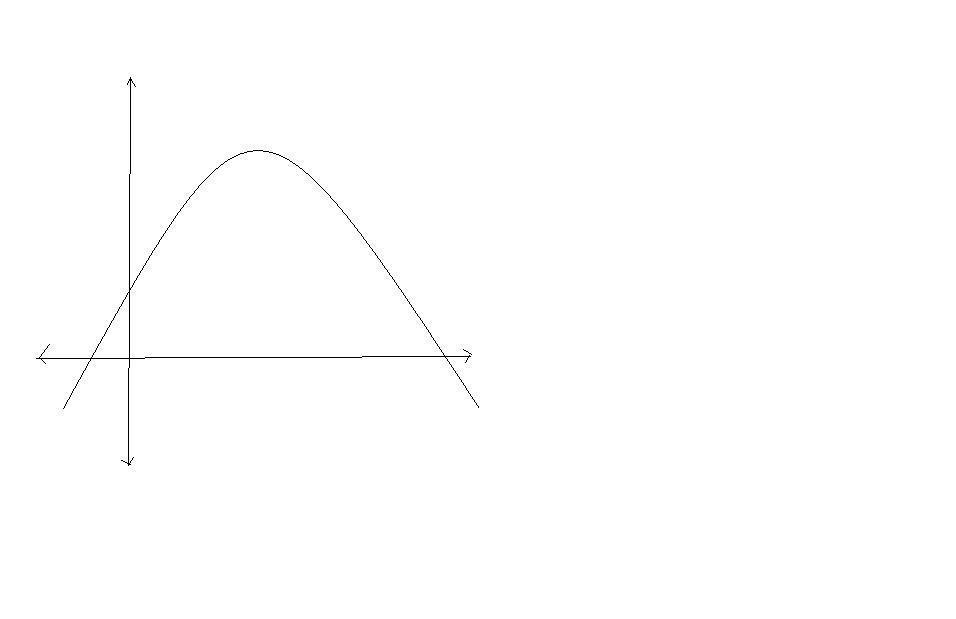$\large 2.$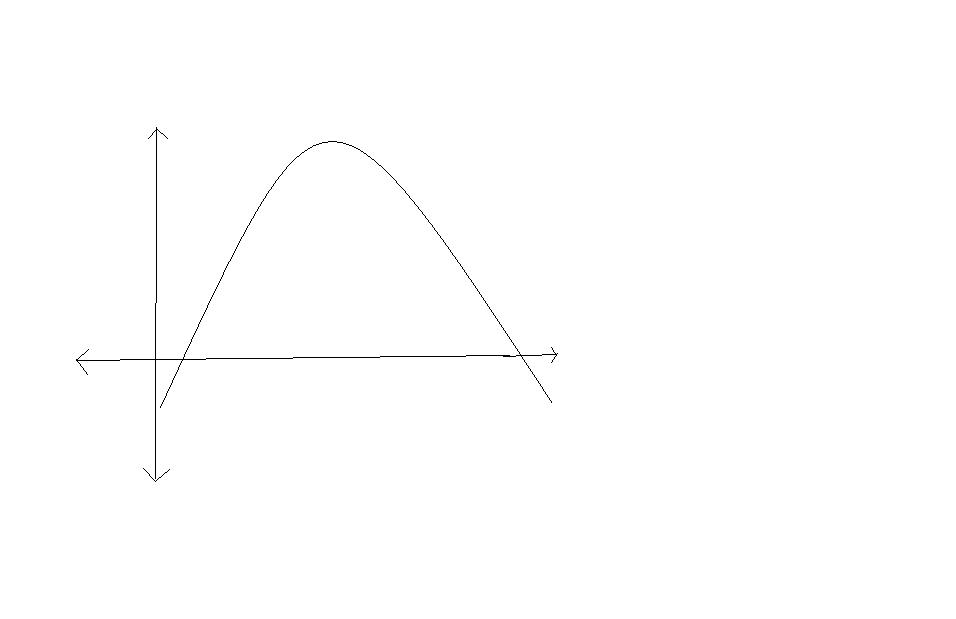$\large 3.$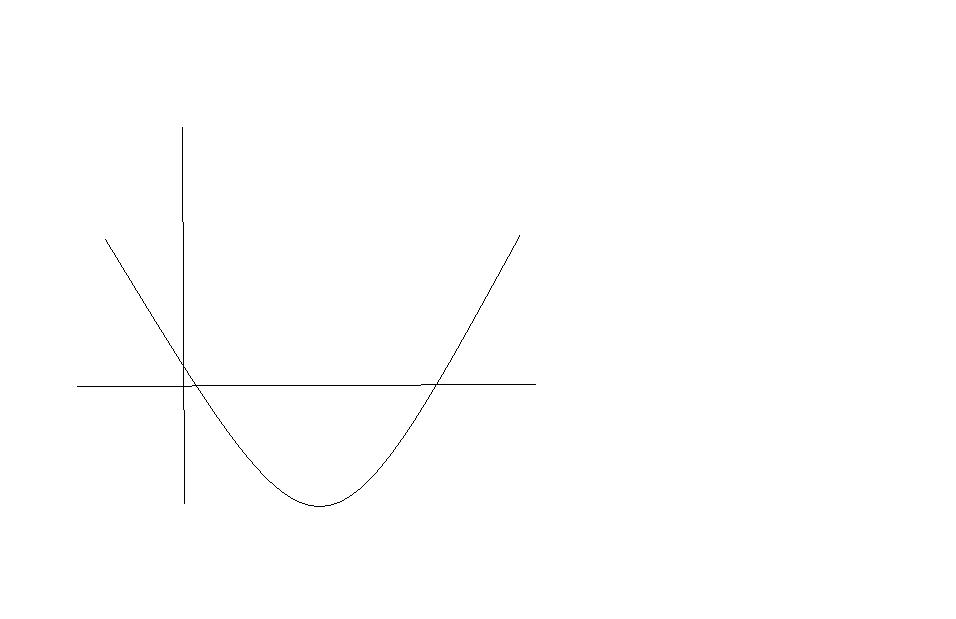$\large 4.$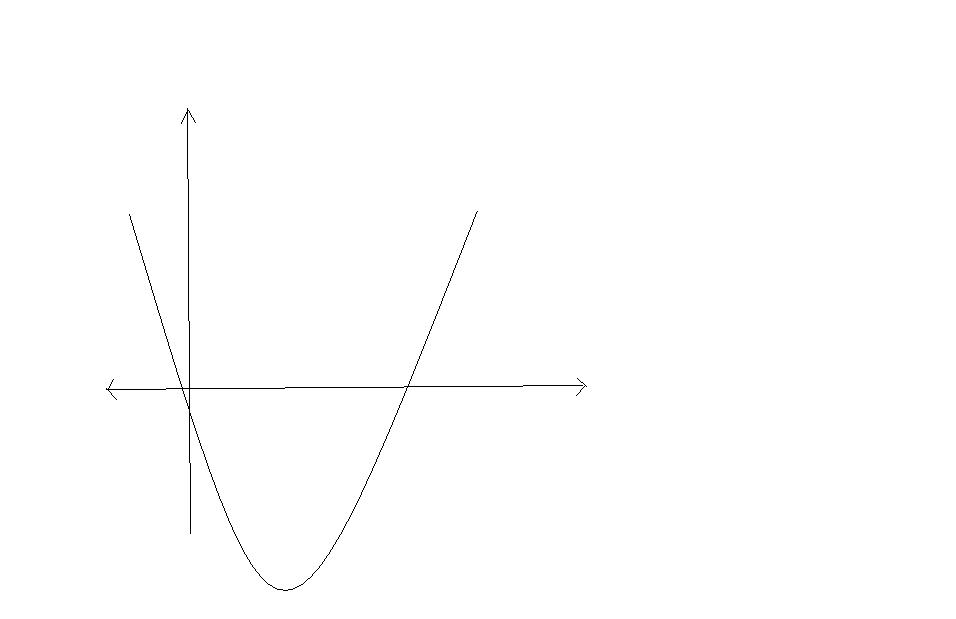$\large 5.$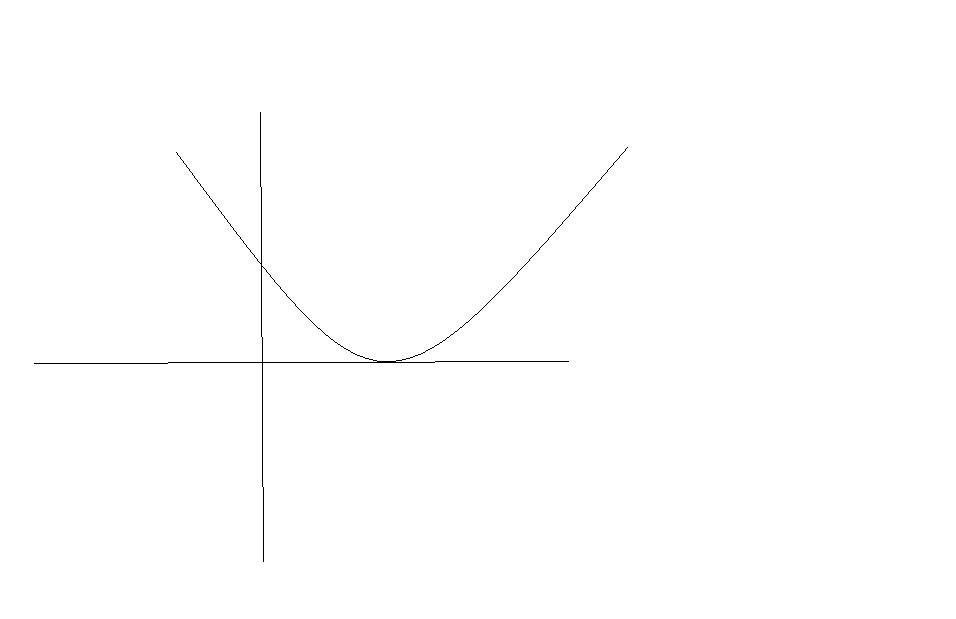×